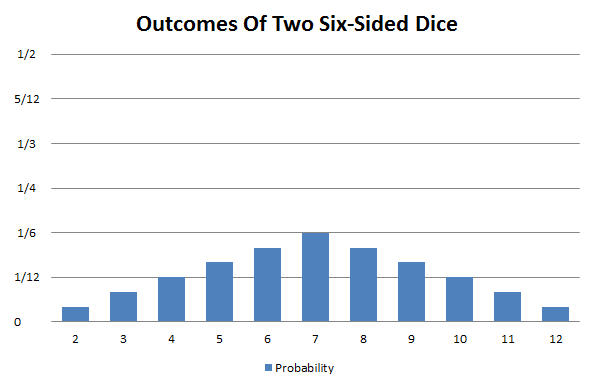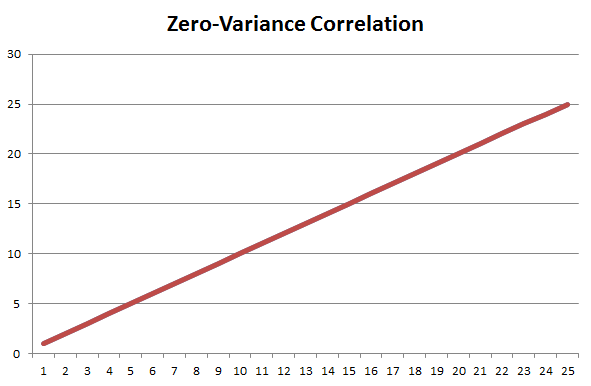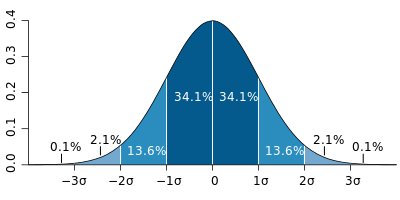# Video Game Statistics: A Primer – Game Planning With Science! Part 3

## By Reading This Post, You’ll Learn:

• The difference between statistics and the real world
• Why we use statistics
• What a probability distribution is, and the difference between discrete and continuous distributions
• The semantic difference between and average and a mean
• The limitation of averages
• How to calculate the spread of a data set using variance and standard deviations
• Lot’s of Excel formulas for the above!

# What Are Statistics?

We live in the real world, and real things happen in the real world for real reasons. Lots of real things, for lots of real reasons. Too many to calculate, in fact. We cannot possibly gather every characteristic of everything. It’s simply too much information to collect or even to understand.

Statistics is the science of simplifying the real world into quantifiable numbers that we can understand. Instead of an entire population (example, every left-handed person on Earth), we take a sample of that population (200 hundred left-handed people). Instead of getting the exact characteristic of that population (there is some true mean lifespan of all the left-handed people on earth), we take the sample and calculate a statistic (the mean life-span of the sample). We then analyze that statistic to make inferences about the original population.

### Why Use Statistics?

Statistical analyses can be quite complicated, but, at a high-level, here are the things statistical analysis is trying to determine:

1. What can we infer about the population from this sample? Example: the mean life span of a left-handed person is 5 years less than a right-handed person.
2. What is the margin of error on this analysis? Example: +/- 7 years.
3. How confident are we that the actual population mean lies within that margin of error? Example: we are 95% confident that the true average lifespan of a left-handed person is between 12 years less and 2 years more than that of a right-handed person.
4. Is the relationship between these variables significant? Example: we can say that there is only a 5% chance that the difference in lifespan between left- and right-handed people in our sample is random coincidence.

Perhaps you knew all of that, intuitively or otherwise. But I find that it’s always important to keep these concepts in mind when looking at data. You are trying to understand the real world by looking at a simplified version of it. Your lack of complete information means that there will always be some margin of error. The size of your margin of error is proportional to your confidence that the true value lies within that margin. In the example above, a smaller margin of error of +/-4 years might only give you 75% confidence that it contains the true value of the population.

And finally, always remember that you, generally speaking, cannot prove that something is true with statistics. You can only demonstrate that it is more likely than something else.

# A Roll Of The Dice: Probability Distributions

Think of your run-of-the-mill, six-sided die. On any give roll, you have exactly 1/6 chance of rolling one of the six values of the die faces. If you were to graph the expected outcomes, along with their probabilities, it would look like this:Image source: the author

What if you rolled two six-sided dice? Your possible outcomes shift from 1 through 6 to 2 through 12 and the probabilities for the individual values change dramatically. Instead of 6 possible rolls, you have 6*6=36 potential outcomes. You have a 1/36 chance of rolling 2 or 12, 1/18 of rolling 3 or 11, 1/12 of 4 or 10, so on and so forth.Image source: the authorImage source: the author

These graphs are examples of probability distributions. A probability distribution is the respective chance of seeing each possible outcome of an experiment or event, with the sum of all of the individual probabilities adding up to 100%. Worded differently, a probability distribution contains every possible outcome, and the odds of seeing any one particular outcome.

### Continuous vs. Discreet Distributions

Probability distributions come in many flavors, but they fall into two broad categories.

First you have discrete distributions. These have a finite number of possible outcomes within the distribution, such as the dice example above. Other examples would be number of children, or number of phone calls place in 24 hours.

There are also continuous distributions. In a continuous distribution, there is an effectively infinite number of possible outcomes, even with a finite range of values. Examples of such distributions: height, weight, or lifespan.

#### In Simple Terms

Basically, a discrete distribution is when an outcome is either one value or another – you either have 2 siblings, or 3, or 4. You don’t have 2.57634343 siblings. A continuous distribution is used when the observations can fall anywhere in the range of outcomes. You might be 5’9″, or 5’9.5″ or 5’9.54458388″, depending on your level of precision.

One of the paradoxes of continuous distributions is that, because there are infinite possible outcomes, there is effectively zero chance of hitting any one value exactly. The probability of an exact value occurring is essentially one divided by infinity. Therefore, when dealing with a continuous distribution, you can only determine the probability of a range of outcomes within the overall distribution: the probability of the outcome being greater than a particular value, less than a particular value, or falling between two particular values.

# The In’s And Out’s Of Averages

Maybe it’s a salience bias thing, but it seems like I’m constantly seeing posts from various outlets about losing weight. And I find this slightly maddening. What these articles (presumably) mean by “weight” is “fat”. But using those two terms interchangeably is counter-productive to your health. Your weight is comprised of lots of materials: fat, yes, but also muscle, bone, fluids, food, and all sorts of other substances that avail one’s body of mass.

If I hop on the scale today and I weigh 0.6 pounds less than yesterday, all I know is that I’ve lost weight. But I have no idea what kind of weight. I very well may have lost 0.6 pounds of muscle, particularly if I’m restricting calories. Changes in weight only have meaning when combined with a complimentary reading, such as a body-fat measurement. Only with that secondary data point can you make a meaningful analysis of how your health has changed.

I feel the same way about averages. Or should I say means, because the word “average” has a different interpretation in statistics. We’re taught in school to think of an average in the arithmetical sense: the sum of a set of values divided by the number of those values. But in statistics, the term “average” means “typical”. As in “The average male is 5’9 and weighs 180 lbs”. Statistics calls the arithmetical average the “mean”. And much like the example about weight loss above, simply calculating the mean of a data set only tells you part of the story and ignores some crucial information.

### Understanding the Limitations of Mean Values: An Example

Imagine that you and I are sitting around and we are just bored out of our minds. So we start making bets on coin flips. Heads or tails? Heads or tails? Over and over (we’re really bored). With each coin flip, we bet more money:

 Flip Bet 1 \$1 2 \$10 3 \$100 4 \$1,000 5 \$10,000

In each case, each of us has a 50/50 chance of winning the bet. Arithmetically speaking, we each have a probability distribution of a 50% chance of a loss and 50% chance of a win. This nets each of us an expected value, in every round, of (.5*Bet-.5*Bet) or \$0. In other words, we have the same mean expected payoff of zero dollars in each and every round.

So, Round 5 is equivalent to Round 1, right? Of course not.

Round 5 carries way more risk. But simply calculating the mean outcome of each round communicates nothing about that risk. It only tells us the expected outcome of the probability distribution.

While Rounds 1 and 5 have the same expected outcome, they have drastically different variances. The mean outcome may be the same, but the probability distribution of Round 5 is significantly wider.

### Not All Distributions Look Alike

Another danger with only calculating the mean lies in how we are taught to think of distributions: as a bell curve. But bell curves are only one flavor of graph. If all you knew of the following distributions was their mean value, think how much information you would be missing:Think how little you would understand about each these distributions if all you knew was their respective means (Source: Wikipedia, public domain image)

The moral of the story: if you are forecasting productivity using only your arithmetical average, you are missing critical information that could drastically impact your planning process. The mean is a crucial value, but it can’t tell you the whole story by itself.

# Embracing Chaos: Understanding Variance

Variance is the measurement of how far your data stray from the mean value. It’s a measurement of “spread”. If all of your data have the same value – if all are equal to the mean – you would have zero variance, and your probability distribution would be a straight vertical line.

Or if there was a perfectly linear correlation between two variables (eg, for every 1 cheeseburger any person eats, that person gains 1 pound of fat), your Cartesian graph would show a straight line with the slope y = x.Image source: the author

However, as soon as there is any variance, your mean changes from a data point in and of itself into an indicator of central tendency. It shows you the value around which your data anchor. But – and this is important to understand – it’s entirely possible that none of your data are actually equal to the mean or would fall on a Cartesian line.

When variance is at play (which it will be with real-world data), your mean is the line of “best fit”. It gives you a sense of order within the chaos, but is not sufficient information on its own.Image source: the author

### Calculating Variance

Calculating variance is super easy if you have data handy: enter your data into Excel, and then use Excel’s “=var.s()” function*. But here’s the rub: the value of your variance isn’t actually meaningful, from an interpretation stand point. I’ll skip the minutiae of the calculations behind variance (you can find them here, if you’re so inclined), but the output returns a squared value.

For instance, if you have a list of how long, in hours, every feature in your game took to code, and plugged that into Excel and used the “=var.s()” function to calculated the variance, the result would be in square hours (eg, 3600H²). And what the hell do you do with a square hour?

### Making Sense of Variance: Standard Deviations

In order to actually interpret your variance, you need to calculate its square root, which is called the standard deviation. In our example above, the a variance of 3600H² yields a standard deviation of 60 hours. Excel also has a standard deviation function, “=stdev.s()”, so you can skip that variance mumbo-jumbo, what with its nonsensical squared units.

Assuming the data you’re analyzing has a typical bell-curve (called a normal distribution in statistics), one of the more useful aspects of a standard deviation is that it can tell you how much data is covered by how wide a probability spread. One standard deviation from the mean in each direction will cover 68.2% of the probability distribution (you can be 68.2% confident an outcome will fall in that range). Two standard deviations will cover 95.4%. Three will cover 99.6%º.

If you want to 99.6% sure that an outcome will fall within a given range (say, at what point in time you can deliver X features), you need to take the expected value (the mean time per feature, times the number of features), and then add 3 standard deviations in either direction from the expected value. I cover how to use the standard deviation to calculate future dates in Part 7 of “Game Planning With Science!”.A graphical depiction of how a normal bell-curve breaks down into standard deviations. (Source: By Mwtoews – Own work, based (in concept) on figure by Jeremy Kemp, on 2005-02-09, CC BY 2.5, used under Creative Commons ShareAlike license, https://commons.wikimedia.org/w/index.php?curid=1903871)

## Further Reading If You Enjoyed This Post

Scheduling Video Games Scientifically

Planning Games Using The Central Limit Theorem

Sunk Costs and Ugly Babies: On the Value of the Scientific Method

# Where Do We Go From Here?

We’re not quite done with statistics yet. The post sets up the next post which will cover one of the most phenomenal concepts in decision sciences: the Central Limit Theorem. Click here to read on!

## Key Takeaways

• Statistics is the science (and, to some extent, art) of trying to understand the complexities of the real world using simplified mathematical approximations
• Real world populations have characteristics; we take a sample of the population and calculate a statistic to estimate the actual value of respective characteristic
• At a high level, a statistical analysis is looking to identify the mean, margin of error, confidence, and significance of the sample statistics
• Probability distributions are all of the possible outcomes of an event or experiment and the respective probabilities of those outcomes
• Probability distributions can be discrete or continuous, but in either case, the sum of all of the individual probabilities is equal to 1
• In statistics, “average” means “typical”; the term for the arithmetic average is “mean”
• Your expected value is the weighted average of the probability distribution.
• The mean alone is only one data point and tells you nothing of the spread of possible outcomes
• Variance is the measure of that spread from the mean, but is calculated in terms of square units and thus might not have a meaningful interpretation by itself
• Standard deviation is the square root of the variance, providing a more meaningful interpretation of the spread of the probability distribution
• In a normal distribution, one standard deviation from the mean encompasses 68.2% of the possible outcomes, two cover 95.4%, and three cover 99.6%.

## Key Excel Formulas

• “=average()” finds the average of all of the data cells you reference in the parentheses
• “=var.s()” finds the variance of all of the data cells you reference in the parentheses
• “=stdev.s()” finds the standard deviation of all of the data cells you reference in the parentheses